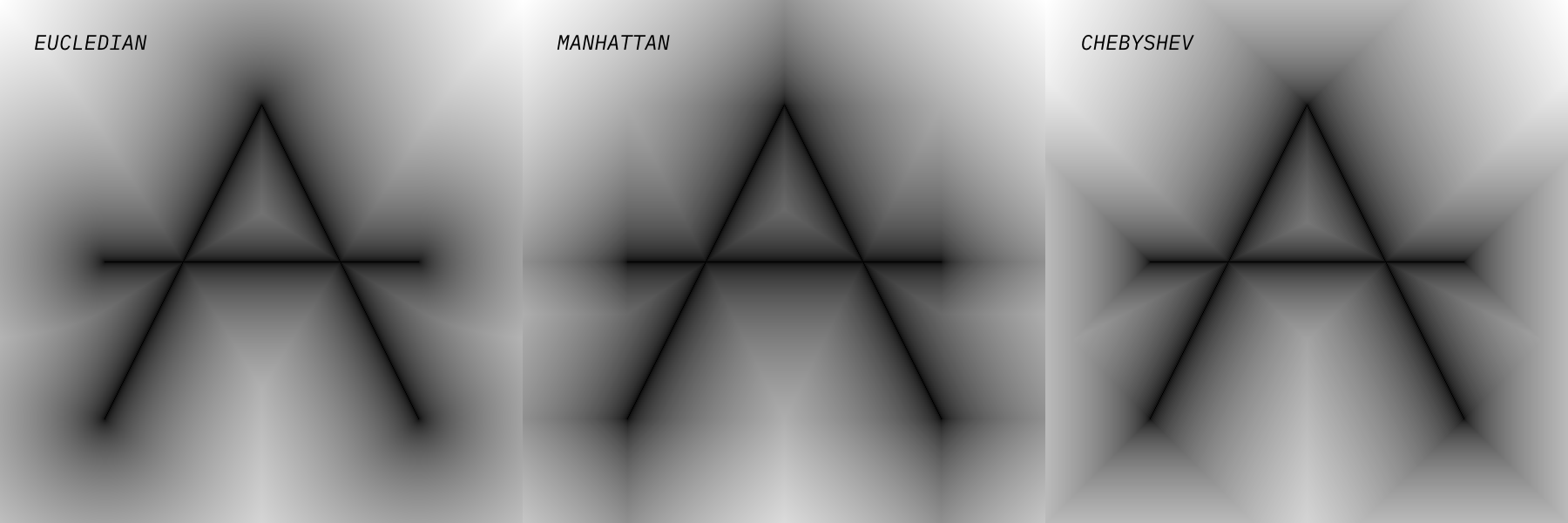Options
All
• Public
• Public/Protected
• AllThis project is part of the @thi.ng/umbrella monorepo.

Binary image to Distance Field transformation.This package provides a function to transform a binary(-like) input grid/image into a distance field using a provided distance metric (default: Eucledian). Any non-zero values in the input grid are used as seed locations for the distance field. The function returns a plain Float32Array of distance values. If normalize is > 0 (default: 1). The result values will be normalized to the [0,normalize] interval.

Based on: "A general algorithm for computing Distance Transforms in linear time", A. Meijster, J.B.T.M. Roerdink and W.H. Hesselink

Status

ALPHA - bleeding edge / work-in-progress

Search or submit any issues for this package

Installation

ES module import:

<script type="module" src="https://cdn.skypack.dev/@thi.ng/distance-transform"></script>

Skypack documentation

For Node.js REPL:

# with flag only for < v16
node --experimental-repl-await

> const distanceTransform = await import("@thi.ng/distance-transform");

Package sizes (gzipped, pre-treeshake): ESM: 658 bytes

API

Generated API docs

This small example uses functionality from the @thi.ng/pixel and @thi.ng/random packages...

import { distanceTransform } from "@thi.ng/distance-transform";
import { floatBuffer, intBuffer, canvas2d, GRAY8, FLOAT_GRAY } from "@thi.ng/pixel";
import { SYSTEM } from "@thi.ng/random";

// create image with 100 random pixels set
const img = intBuffer(256, 256, GRAY8);
for(let i = 0; i < 100; i++) {
img.setAt(SYSTEM.int() % img.width, SYSTEM.int() % img.height, 255);
}

// compute distance field (aka voronoi)
const dt = distanceTransform(img, EUCLEDIAN);

// wrap as float pixel buffer
const dtImg = floatBuffer(img.width, img.height, FLOAT_GRAY, dt);

// ...and display (browser only)
const { canvas } = canvas2d(img.width, img.height, document.body);
dtImg.blitCanvas(canvas);

Authors

Karsten Schmidt

If this project contributes to an academic publication, please cite it as:

@misc{thing-distance-transform,
title = "@thi.ng/distance-transform",
author = "Karsten Schmidt",
note = "https://thi.ng/distance-transform",
year = 2021
}